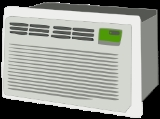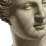xAir conditioning
Posts  1 - 1  of  1behmin
How do you calculate power for a compressor with the following conditions theoretically? Does the power change with different suction superheats? You can assume issentropic efficiency of 70%. Thanks.

Condition 1.
Refrigerant = R410A
Psuction = 105 psig
Tsuction = 44 deg F
Pdischarge = 330 psig

Condition 2.
Refrigerant = R410A
Psuction = 105 psig
Tsuction = 64 deg F
Pdischarge = 330 psig